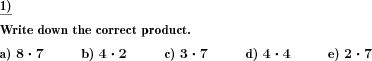Custom math worksheets at your fingertips# Details for problem "Times table:Multiplication problems in range one to ten"

Quickname: 7354

Elementary School, Primary School, Middle School.

## Summary

Multiplication problems from the 1-10 times tables.

## Example## Description

Use these very differentiated problem to help learners in boosting their times table reading skills. In this task, multiplication problems have to be solved. These are taken from the multiplication tables. All multiplication tables from 1 to 10 can be the subject of the task at the same time or only a selected row.

This problem are a great resource to enhance the timetables skills of grades 3 to 5 by using in group activities, quick learning check, independent learning, or homework practice. The practice of this worksheet may help in achieving the following objectives.

1. Revise all times table learned.
2. Develop strong concepts and fluency of the times table.
3. Build up the concrete foundation for mathematical concepts.
4. Calculate mathematical statements for multiplication within the multiplication tables and write them using the multiplication (×), and equals (=) signs.
5. Show that multiplication of two numbers can be done in any order (commutative).
6. Solve problems involving multiplication using repeated addition and mental methods.

Generate a personalized worksheet based on the activity type and requirements of learners. Accordingly, both factors from the range of one to ten are selected, or only one of the factors, while the other factor remains fixed according to the selected table.

The number of problems set is adjustable from 1 to 10.

Download free printable worksheets for this math problem here. The worksheet contains the problems only, the solution sheet includes the answers. Just click on the respective link.

•Worksheet 1Solution sheet with answers
•Worksheet 2Solution sheet with answers
•Worksheet 3Solution sheet with answers

If you can not see the solution sheets for download, they may be filtered out by an ad blocker that you may have installed. If this is the case, please allow ads for this page and reload the page. The solution sheets will then reappear.

• Do these sample worksheets do not really fit?
• Do you need more math worksheets, with a different level of difficulty?
• Would you like to combine different problems on a worksheet and adjust them to your needs?
• As a teacher, you can put together your own worksheets using the automatically generated math problems provided.
With a free initial credit, you can start creating your own math worksheets in a few minutes.

You can try it for free! Register here, to create custom worksheets now!

## Customization options for this problem

Parameter
Possible values
Number of problems
1, 2, 3, 4, 5, 6, 7, 8, 9, 10
Number to train
all, 1, 2, 3, 4, 5, 6, 7, 8, 9, 10

## Similar problems

Remark
Description
As a division problem
Division problems from the multiplication tables
A series of multiplication problems, introducing a factor of ten
Multiplication problem series introducing factor of ten